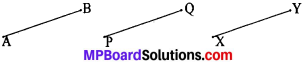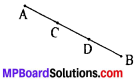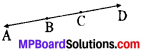# MP Board Class 9th Maths Solutions Chapter 5 Introduction to Euclid’s Geometry Ex  5.1

## MP Board Class 9th Maths Solutions Chapter 5 Introduction to Euclid’s Geometry Ex  5.1

Question 1.
Which of the following statements are true and which are false? Give reasons for your answers.

1. Only one line can pass through a single point.
2. There are infinite number of lines which pass through two distinct points.
3. A terminated line can be produced indefinitely on both the sides.
4. If two circles are equal, then their radii are equal.
5. In Fig. below, if AB = PQ and PQ = XY, then AB = XY.Solution:

1. False, infinitely many lines can pass through a given point.
2. False, only one line can pass through two distinct points.
3. True, by postulate 2 i.e., a terminated line can be produced indefinitely.
4. True, equal circles coincide each other. Therefore their radii will be equal.
5. True, by Euclid’s axiom 1, i.e., things which are equal to the same thing are equal to one another.Question 2.
Give a definition for each of the following terms. Are there other terms that need to be defined first? What are they, and how might you define them?

1. parallel lines
2. perpendicular lines
3. line segment
5. square

Solution:
1. Parallel lines:
Two distinct lines in a plane are called parallel lines if they do not have a common point. Here the undefined terms are lines and plane.

2. Perpendicular lines:
Two lines are perpendicular to each other if they intersect each other at right angle. Here the undefined term is right angle.

3. Line segment:
A part of a line between two points on a line is called a line segment. Here the undefined term is part of a line.

Radius of a circle is the distance of a point on the circle from the center of the circle.

5. Square:
A square is a rectangle having all sides equal. Here undefined term is rectangle.

Question 3.
Consider two ‘postulates’ given below:

1. Given any two distinct points A and B, there exist a third point C which is in between A and B.
2. There exist at least three points that are not on the same line.

Do these postulates contain any undefined terms? Are these postulates consistent? Do they follow from Euclid’s postulates? Explain.
Solution:
Yes, these postulates contain undefined terms such as point, line, distinct points. They are consistent because they deal with two different situations:

1. Point C is lying between two distinct points A and B on a line.
2. Point C is not lying on the line through A and B.

These postulates do not follow from Euclid’s postulates. However they follow from axiom “given two distinct points, there is a unique line that passes through them.Question 4.
If a point C lies between two points A and B such that AC = BC, then prove that AC = $$\frac{1}{2}$$AB. Explain by drawing the figure.
Solution:
Given: AC = BC
To prove: AC = $$\frac{1}{2}$$AB
Proof:
AC = BC
AC + AC = BC + AC
2AC = AB
AC = $$\frac{1}{2}$$AB

Question 5.
In Question 4, point C is called a mid-point of line segment AB. Prove that every line segment has one and only one midpoint.
Solution:
If possible, Let us assume that a line segment AB has two mid points C and D when C is the mid point of ABAC = 1/2 AB …(i)
where D is the mid point of AB
From (i) and (ii), we get
(By Euclid’s axiom, things which are half of the same thing are equal to one another.). This is possible only if C and D coincides.
∴ Our assumption that C and D are two mid points of AB are wrong and hence a line segment has one and only one mid point.

Question 6.
In Fig. below, if AC = BD, then prove that AB = CD.Solution:
Given: AC = BD
To prove: AB = CD
Proof:
AC = BD
Subtracting BC on both sides, we get
AC – BC = BD – BC (By Euclid’s axiom-3)
∴ AB = CDQuestion 7.
Why is Axiom 5, in the list of Euclid’s axioms, considered a ‘universal truth’? (Note that the question is not about the fifth postulate).
Solution:
We know that whole is always greater than its part.